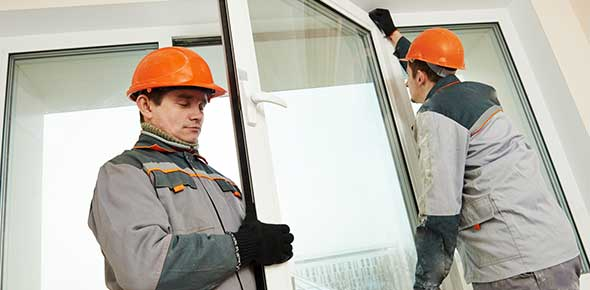# Physical Processes - Friction

10 Questions | Total Attempts: 1560Settings• 1.
A puck slides along an icy surface. What makes it slow down?
• A.

It runs out of push

• B.

It rubs against the ice

• C.

It runs out of electricity

• 2.
A boy sits halfway down a grassy slope. What force stops him sliding down?
• A.

Weight

• B.

Gravity

• C.

Friction

• 3.
On which surface will a toy sledge travel the furthest?
• A.

Carpet

• B.

Polished wood

• C.

Ice

• 4.
On which surface will a toy sledge travel the least distance?
• A.

Carpet

• B.

Polished wood

• C.

Ice

• 5.
The rougher the surface, ...
• A.

The greater the friction

• B.

The less the friction

• C.

The same the level of friction

• 6.
Which of the following is an example of trying to increase friction?
• A.

Squirting oil into door hinges

• B.

• C.

Waxing the underneath of skis

• 7.
Which of the following statements is true?
• A.

Friction is a force that only occurs between solids

• B.

Friction is a force that only occurs on rough surfaces

• C.

Friction is a force that only occurs when surfaces touch each other

• 8.
Which of the following statements is false?
• A.

Friction can only slow things down

• B.

Friction is never useful

• C.

Friction can stop things from moving

• 9.
Air resistance and water resistance are both types of friction. Is this true or false?
• A.

True

• B.

False

• C.

Neither true nor false

• 10.
If you poured oil onto a wooden surface, the friction would ...
• A.

Be reduced

• B.

Be increased

• C.

Remain the same

Related TopicsBack to top